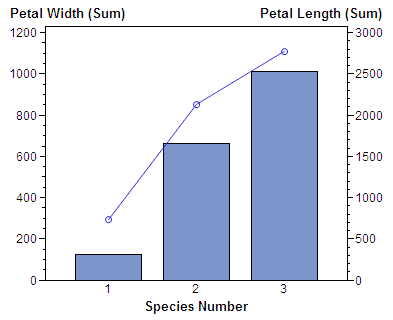# Overview: GBARLINE Procedure

## About the GBARLINE Procedure

The GBARLINE procedure produces bar-line charts. Bar-line charts are vertical bar charts with one or more plot overlays. These charts graphically represent the value of a statistic calculated for one or more variables in an input SAS data set. The charted variables can be either numeric or character.
The procedure calculates these statistics:
• sum
• mean
• frequency or cumulative frequency
• percentage or cumulative percentage
Use the GBARLINE procedure to do the following tasks:
• display and compare exact and relative magnitudes
• examine the contribution of parts to the whole
• analyze where data are out of balance
• display a long series of data, and show trends and patterns
In conjunction with the SYMBOL statement, the GBARLINE procedure can produce needle plot overlays, and overlay plots with stepped interpolation.
Note: PROC GBARLINE is not supported by Java.

## Chart Terminology

Bar-line charts display the magnitude of data with bars, each of which represents a category of data (midpoint). The height of the bars represents the value of the bar statistic for the corresponding midpoint.
Bar-Line Chart shows the relationship between petal width and petal length for three species of flowers. The horizontal axis is the midpoint axis and the vertical axes are response axes. The right response axis is the PLOT statement axis and the left vertical axis is the BAR statement axis. Each axis is labeled with the variable name or label. Each species is a midpoint, so each bar is labeled with the species identifier.
Bar-Line Chart